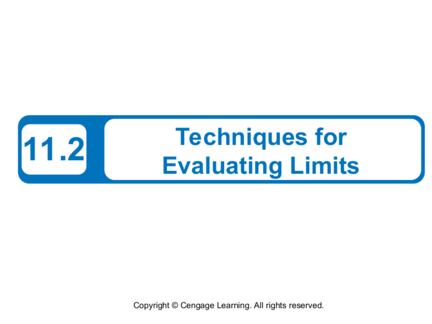# Techniques for Evaluating LimitsContributed by:LEARNING OUTCOMES:
1. Use the dividing out technique to evaluate limits of functions.
2. Use the rationalizing technique to evaluate the limits of functions.
3. Use technology to approximate the limits of functions graphically and numerically.
4. Evaluate one-sided limits of functions 5. Evaluate limits of difference quotients from calculus.
1. Techniques for
11.2 Evaluating Limits
2. What You Should Learn
• Use the dividing out technique to evaluate limits
of functions
• Use the rationalizing technique to evaluate
limits of functions
• Use technology to approximate limits of
functions graphically and numerically
2
3. What You Should Learn
• Evaluate one-sided limits of functions
• Evaluate limits of difference quotients from
calculus
3
4. Dividing Out Technique
4
5. Dividing Out Technique
We have studied several types of functions whose limits
can be evaluated by direct substitution.
In this section, you will study several techniques for
evaluating limits of functions for which direct substitution
Suppose you were asked to find the following limit.
5
6. Dividing Out Technique
Direct substitution fails because –3 is a zero of the
denominator. By using a table, however, it appears that the
limit of the function as x approaches –3 is –5.
6
7. Example 1 – Dividing Out Technique
Find the limit.
Begin by factoring the numerator and dividing out any
common factors.
Factor numerator.
7
8. Example 1 – Solution cont’d
Divide out common
factor.
(x – 2) Simplify.
= –3 – 2 Direct substitution
= –5 Simplify.
8
9. Dividing Out Technique
This procedure for evaluating a limit is called the dividing
out technique.
The validity of this technique stems from the fact that when
two functions agree at all but a single number c, they must
have identical limit behavior at x = c.
In Example 1, the functions given by
f (x) and g (x) = x – 2
agree at all values of x other than x = –3.
So, you can use g (x) to find the limit of f (x).
9
10. Dividing Out Technique
The dividing out technique should be applied only when
direct substitution produces 0 in both the numerator and
the denominator.
An expression such as has no meaning as a real
It is called an indeterminate form because you cannot,
from the form alone, determine the limit.
10
11. Dividing Out Technique
When you try to evaluate a limit of a rational function by
direct substitution and encounter this form, you can
conclude that the numerator and denominator must have a
common factor.
After factoring and dividing out, you should try direct
substitution again.
11
12. Rationalizing Technique
12
13. Rationalizing Technique
Another way to find the limits of some functions is first to
rationalize the numerator of the function. This is called the
rationalizing technique.
We have known that rationalizing the numerator means
multiplying the numerator and denominator by the
conjugate of the numerator.
For instance, the conjugate of + 4 is – 4.
13
14. Example 3 – Rationalizing Technique
Find the limit.
By direct substitution, you obtain the indeterminate form .
Indeterminate form
14
15. Example 3 – Solution cont’d
In this case, you can rewrite the fraction by rationalizing the
Multiply.
Simplify.
15
16. Example 3 – Solution cont’d
Divide out common factor.
Simplify.
,x≠0
Now you can evaluate the limit by direct substitution.
16
17. Example 3 – Solution cont’d
You can reinforce your conclusion that the limit is by
constructing a table, as shown below, or by sketching a
graph, as shown in Figure 11.12.
Figure 11.12
17
18. One-Sided Limits
18
19. One-Sided Limits
The limit of f (x) as x  c does not exist when the function
f (x) approaches a different number from the left side of c
than it approaches from the right side of c.
This type of behavior can be described more concisely with
the concept of a one-sided limit.
f (x) = L1 or f (x)  L1 as x  c– Limit from the left
Limit from the right
f (x) = L2 or f (x)  L2 as x  c+
19
20. Example 6 – Evaluating One-Sided Limits
Find the limit as x  0 from the left and the limit as x  0
from the right for
f (x) = .
From the graph of f, shown in
Figure 11.15, you can see
that f (x) = –2 for all x < 0.
Figure 11.15
20
21. Example 6 – Solution cont’d
So, the limit from the left is
Limit from the left
= –2.
Because f (x) = 2 for all x > 0, the limit from the right is
= 2. Limit from the right
21
22. One-Sided Limits
22
23. A Limit from Calculus
23
24. A Limit from Calculus
A Limit from Calculus In the next section, you will study an
important type of limit from calculus—the limit of a
difference quotient.
24
25. Example 9 – Evaluating a Limit from Calculus
For the function given by f (x) = x2 – 1, find
Direct substitution produces an indeterminate form.
25
26. Example 9 – Solution cont’d
By factoring and dividing out, you obtain the following.
26
27. Example 9 – Solution cont’d
= (6 + h)
=6+0
=6
So, the limit is 6.
27